Currency Strength Score/Index formula11613

Hi,

I'm looking to make an EA works with multiple currencies, there's a lot of indicator that show a meter based on a score or a index. Unfortunately, none of them's code is available. I've googled to find the formula, but ... didn't find nothing.

Anyone knows such a formula please ?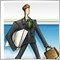4749

blouf:

Hi,

I'm looking to make an EA works with multiple currencies, there's a lot of indicator that show a meter based on a score or a index. Unfortunately, none of them's code is available. I've googled to find the formula, but ... didn't find nothing.

Anyone knows such a formula please ?

see this: https://www.mql5.com/en/forum/16365

file attached.

Files: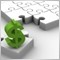8798

I prefer to use "raw" currency strength rather than abstract values that some currency strength indicators use. And it's simple. You simply add up all moves in each currency pair. This is normally done on the 8 major currencies (28 pairs).

So to get say AUD's current strength you would look at each pair AUD is in and see how much it has moved (in percentage terms) from open to current price. Remember that a positive or negative move will depend on if the AUD is the base or quote of a pair. So the (exaggerated) calculation would look something like this:

AUDCHF = 1.8%
AUDJPY = 2.5%
AUDNZD = 1.2%
AUDUSD = 1.6%
EURAUD = -2.3% (so + 2.3%)
GBPAUD = -1.9% (so +1.9%)

Total = 13.5%

You don't then divide it by 7 to get an average. You want the raw strength and 13.5% is it. That is the current strength of the Aussie compared to the other 7 currencies. You now do the same calculation for each of the other currencies.

If you've done it correctly, when you add up all 8 currencies, the final answer should be......zero! Why? Currency correlation :)

You can then display the results as either a meter, or as I prefer, a line study. This way you can see the change over time and rate of change to see which is gaining strength, which is losing strength and the speed or strength of the change. So you end up with something like this: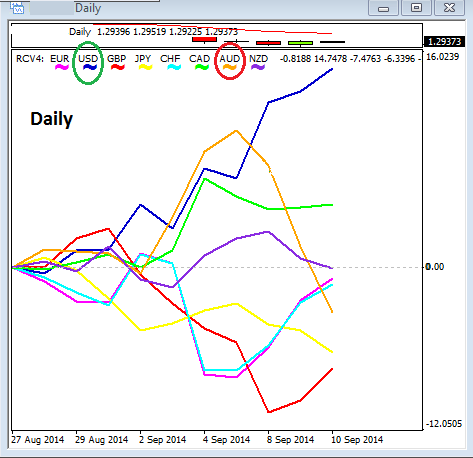</Rant>  :)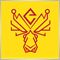6

Thanks for the clear explanation Stuart! :)173

Stuart Browne:

I prefer to use "raw" currency strength rather than abstract values that some currency strength indicators use. And it's simple. You simply add up all moves in each currency pair. This is normally done on the 8 major currencies (28 pairs).

So to get say AUD's current strength you would look at each pair AUD is in and see how much it has moved (in percentage terms) from open to current price. Remember that a positive or negative move will depend on if the AUD is the base or quote of a pair. So the (exaggerated) calculation would look something like this:

AUDCHF = 1.8%
AUDJPY = 2.5%
AUDNZD = 1.2%
AUDUSD = 1.6%
EURAUD = -2.3% (so + 2.3%)
GBPAUD = -1.9% (so +1.9%)

Total = 13.5%

You don't then divide it by 7 to get an average. You want the raw strength and 13.5% is it. That is the current strength of the Aussie compared to the other 7 currencies. You now do the same calculation for each of the other currencies.

If you've done it correctly, when you add up all 8 currencies, the final answer should be......zero! Why? Currency correlation :)

You can then display the results as either a meter, or as I prefer, a line study. This way you can see the change over time and rate of change to see which is gaining strength, which is losing strength and the speed or strength of the change. So you end up with something like this:

</Rant>  :)

What method did you use to draw these lines? I'm having a difficult time figuring out how to draw the lines based on the customly calculated numbers.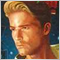568

Stuart is calculating and plotting this within indicator https://www.mql5.com/en/docs/customind173

Enrique Dangeroux:
Stuart is calculating and plotting this within indicator https://www.mql5.com/en/docs/customind

Thank you that was a helpful article. I'm still running into some serious problems getting my lines to draw correctly. They all draw flat. I'm stuck. I dont know how to get the lines to use my calculation on each candle. For some reason its using the same value on each candle. I'm reluctant to post the code here since its a MQL4 issue.173

I dont suppose someone could help me figure out why my lines are drawing backwards? You can see in the top photo my graph and you can see in the bottom photo and in stuarts photo that my lines are forming backwards from how they should be. they should originate at the lookback and widen towards the current candle. Can someone please show me what I am doing wrong?

//+------------------------------------------------------------------+
//|                               My_Currency_Strength_Indicator.mq4 |
//|                                               Erick_Fenstermaker |
//|                                             https://www.mql5.com |
//+------------------------------------------------------------------+
#property version   "1.00"
#property strict

//Opening a separate window and setting the parameters of the window extents
#property indicator_separate_window
#property indicator_maximum 3
#property indicator_minimum -3
#property indicator_level1 0

//Setting the number of memory buffers
#property indicator_buffers 8

//Setting up the memory allocation and default settings for the indicator lines
#property indicator_color1 clrPurple
#property indicator_color2 clrRed
#property indicator_color3 clrOrange
#property indicator_color4 clrWhite
#property indicator_color5 clrBlue
#property indicator_color6 clrGreen
#property indicator_color7 clrLightBlue
#property indicator_color8 clrYellow

//setting width of lines
#property indicator_width1 2
#property indicator_width2 2
#property indicator_width3 2
#property indicator_width4 2
#property indicator_width5 2
#property indicator_width6 2

//Declaring the array that will draw the line for the indicator
double EUR_Band[];
double GBP_Band[];
double AUD_Band[];
double NZD_Band[];
double USD_Band[];
double CHF_Band[];
double JPY_Band[];

//Declaration of the Arrays to store past values (Base Value)
double lookBackEURGBP[];
double lookBackEURAUD[];
double lookBackEURNZD[];
double lookBackEURUSD[];
double lookBackEURCHF[];
double lookBackEURJPY[];

double lookBackGBPAUD[];
double lookBackGBPNZD[];
double lookBackGBPUSD[];
double lookBackGBPCHF[];
double lookBackGBPJPY[];

double lookBackAUDNZD[];
double lookBackAUDUSD[];
double lookBackAUDCHF[];
double lookBackAUDJPY[];

double lookBackNZDUSD[];
double lookBackNZDCHF[];
double lookBackNZDJPY[];

double lookBackUSDCHF[];
double lookBackUSDJPY[];

double lookBackCHFJPY[];

//Declaration of Arrays to store the current value of the EUR Symbols
double EURGBP[];
double EURAUD[];
double EURNZD[];
double EURUSD[];
double EURCHF[];
double EURJPY[];

double GBPAUD[];
double GBPNZD[];
double GBPUSD[];
double GBPCHF[];
double GBPJPY[];

double AUDNZD[];
double AUDUSD[];
double AUDCHF[];
double AUDJPY[];

double NZDUSD[];
double NZDCHF[];
double NZDJPY[];

double USDCHF[];
double USDJPY[];

double CHFJPY[];

//Declaration of Arrays to store percent change from Base value to the Current Value.
double perEURGBP[];
double perEURAUD[];
double perEURNZD[];
double perEURUSD[];
double perEURCHF[];
double perEURJPY[];

double perGBPAUD[];
double perGBPNZD[];
double perGBPUSD[];
double perGBPCHF[];
double perGBPJPY[];

double perAUDNZD[];
double perAUDUSD[];
double perAUDCHF[];
double perAUDJPY[];

double perNZDUSD[];
double perNZDCHF[];
double perNZDJPY[];

double perUSDCHF[];
double perUSDJPY[];

double perCHFJPY[];

//Declaration of Array to store the true strength of the EUR
double perEUR[];
double perGBP[];
double perAUD[];
double perNZD[];
double perUSD[];
double perCHF[];
double perJPY[];

//Sets how far you look into the past (15 min incremints 4 = 1hr)
int lookBack = 16 + 1;
int timeframe = PERIOD_M15;

//+------------------------------------------------------------------+
//| Custom indicator initialization function                         |
//+------------------------------------------------------------------+

int OnInit()
{
IndicatorBuffers(100);

//--- indicator buffers for drawing the EUR oscillator line
SetIndexBuffer(0,EUR_Band);
SetIndexStyle(0,DRAW_LINE);

SetIndexLabel(0,"EUR Band: ");

//--- indicator buffers for drawing the GBP oscillator line
SetIndexBuffer(1,GBP_Band);
SetIndexStyle(1,DRAW_LINE);
SetIndexLabel(1,"GBP Band: ");

//--- indicator buffers for drawing the AUD oscillator line
SetIndexBuffer(2,AUD_Band);
SetIndexStyle(2,DRAW_LINE);
SetIndexLabel(2,"AUD Band: ");

//--- indicator buffers for drawing the NZD oscillator line
SetIndexBuffer(3,NZD_Band);
SetIndexStyle(3,DRAW_LINE);
SetIndexLabel(3,"NZD Band: ");

//--- indicator buffers for drawing the USD oscillator line
SetIndexBuffer(4,USD_Band);
SetIndexStyle(4,DRAW_LINE);
SetIndexLabel(4,"USD Band: ");

//--- indicator buffers for drawing the CAD oscillator line
SetIndexStyle(5,DRAW_LINE);

//--- indicator buffers for drawing the CHF oscillator line
SetIndexBuffer(6,CHF_Band);
SetIndexStyle(6,DRAW_LINE);
SetIndexLabel(6,"CHF Band: ");

//--- indicator buffers for drawing the JPY oscillator line
SetIndexBuffer(7,JPY_Band);
SetIndexStyle(7,DRAW_LINE);
SetIndexLabel(7,"JPY Band: ");

//--- Indicator buffers for EUR Symbol close values from the past (Base Values)
SetIndexBuffer(8,lookBackEURGBP);
SetIndexBuffer(9,lookBackEURAUD);
SetIndexBuffer(10,lookBackEURNZD);
SetIndexBuffer(11,lookBackEURUSD);
SetIndexBuffer(13,lookBackEURCHF);
SetIndexBuffer(14,lookBackEURJPY);

//--- Indicator buffers for GBP Symbol close values from the past (Base Values)
SetIndexBuffer(15,lookBackGBPAUD);
SetIndexBuffer(16,lookBackGBPNZD);
SetIndexBuffer(17,lookBackGBPUSD);
SetIndexBuffer(19,lookBackGBPCHF);
SetIndexBuffer(20,lookBackGBPJPY);

//--- Indicator buffers for AUD Symbol close values from the past (Base Values)
SetIndexBuffer(21,lookBackAUDNZD);
SetIndexBuffer(22,lookBackAUDUSD);
SetIndexBuffer(24,lookBackAUDCHF);
SetIndexBuffer(25,lookBackAUDJPY);

//--- Indicator buffers for NZD Symbol close values from the past (Base Values)
SetIndexBuffer(26,lookBackNZDUSD);
SetIndexBuffer(28,lookBackNZDCHF);
SetIndexBuffer(29,lookBackNZDJPY);

//--- Indicator buffers for USD Symbol close values from the past (Base Values)
SetIndexBuffer(31,lookBackUSDCHF);
SetIndexBuffer(32,lookBackUSDJPY);

//--- Indicator buffers for CAD Symbol close values from the past (Base Values)

//--- Indicator buffers for CHF Symbol close values from the past (Base Values)
SetIndexBuffer(35,lookBackCHFJPY);

//--- indicator buffers for the current EUR Symbol Ask Values
SetIndexBuffer(36,EURGBP);
SetIndexBuffer(37,EURAUD);
SetIndexBuffer(38,EURNZD);
SetIndexBuffer(39,EURUSD);
SetIndexBuffer(41,EURCHF);
SetIndexBuffer(42,EURJPY);

//--- indicator buffers for the current GBP Symbol Ask Values
SetIndexBuffer(43,GBPAUD);
SetIndexBuffer(44,GBPNZD);
SetIndexBuffer(45,GBPUSD);
SetIndexBuffer(47,GBPCHF);
SetIndexBuffer(48,GBPJPY);

//--- indicator buffers for the current AUD Symbol Ask Values
SetIndexBuffer(49,AUDNZD);
SetIndexBuffer(50,AUDUSD);
SetIndexBuffer(52,AUDCHF);
SetIndexBuffer(53,AUDJPY);

//--- indicator buffers for the current NZD Symbol Ask Values
SetIndexBuffer(54,NZDUSD);
SetIndexBuffer(56,NZDCHF);
SetIndexBuffer(57,NZDJPY);

//--- indicator buffers for the current USD Symbol Ask Values
SetIndexBuffer(59,USDCHF);
SetIndexBuffer(60,USDJPY);

//--- indicator buffers for the current CAD Symbol Ask Values

//--- indicator buffers for the current CHF Symbol Ask Values
SetIndexBuffer(63,CHFJPY);

//--- indicator buffers for the percent change from the past open till the current ask of the EUR values
SetIndexBuffer(64,perEURGBP);
SetIndexBuffer(65,perEURAUD);
SetIndexBuffer(66,perEURNZD);
SetIndexBuffer(67,perEURUSD);
SetIndexBuffer(69,perEURCHF);
SetIndexBuffer(70,perEURJPY);

//--- indicator buffers for the percent change from the past open till the current ask of the GBP values
SetIndexBuffer(71,perGBPAUD);
SetIndexBuffer(72,perGBPNZD);
SetIndexBuffer(73,perGBPUSD);
SetIndexBuffer(75,perGBPCHF);
SetIndexBuffer(76,perGBPJPY);

//--- indicator buffers for the percent change from the past open till the current ask of the AUD values
SetIndexBuffer(77,perAUDNZD);
SetIndexBuffer(78,perAUDUSD);
SetIndexBuffer(80,perAUDCHF);
SetIndexBuffer(81,perAUDJPY);

//--- indicator buffers for the percent change from the past open till the current ask of the NZD values
SetIndexBuffer(82,perNZDUSD);
SetIndexBuffer(84,perNZDCHF);
SetIndexBuffer(85,perNZDJPY);

//--- indicator buffers for the percent change from the past open till the current ask of the USD values
SetIndexBuffer(87,perUSDCHF);
SetIndexBuffer(88,perUSDJPY);

//--- indicator buffers for the percent change from the past open till the current ask of the CAD values

//--- indicator buffers for the percent change from the past open till the current ask of the CHF values
SetIndexBuffer(91,perCHFJPY);

//--- indicator buffers for the true strength of the symbol
SetIndexBuffer(92,perEUR);
SetIndexBuffer(93,perGBP);
SetIndexBuffer(94,perAUD);
SetIndexBuffer(95,perNZD);
SetIndexBuffer(96,perUSD);
SetIndexBuffer(98,perCHF);
SetIndexBuffer(99,perJPY);

//---
return(INIT_SUCCEEDED);
}

//+------------------------------------------------------------------+
//| Custom indicator iteration function                              |
//+------------------------------------------------------------------+

int OnCalculate(const int rates_total,
const int prev_calculated,
const datetime &time[],
const double &open[],
const double &high[],
const double &low[],
const double &close[],
const long &tick_volume[],
const long &volume[],

//if(ArrayGetAsSeries(EUR_Band))
//      Print("EUR_Band[] is timeseries");
//   else
//   ArraySetAsSeries(EUR_Band, true);
//      Print("EUR_Band[] is not timeseries!!!");

//looking at older bars
int counted_bars,limit;
counted_bars = IndicatorCounted();
if(!counted_bars)limit = Bars;// - 2;
else limit = Bars - counted_bars;// - 1;
if(rates_total!=prev_calculated)
for(int i=1; i <= lookBack && i >= 1; i++)
{

//Gathers the EUR Symbol close values from the past (Base Values)
lookBackEURGBP[i] = iClose("EURGBP",timeframe,i);
lookBackEURAUD[i] = iClose("EURAUD",timeframe,i);
lookBackEURNZD[i] = iClose("EURNZD",timeframe,i);
lookBackEURUSD[i] = iClose("EURUSD",timeframe,i);
lookBackEURCHF[i] = iClose("EURCHF",timeframe,i);
lookBackEURJPY[i] = iClose("EURJPY",timeframe,i);

//Gathers the GBP Symbol close values from the past (Base Values)
lookBackGBPAUD[i] = iClose("GBPAUD",timeframe,i);
lookBackGBPNZD[i] = iClose("GBPNZD",timeframe,i);
lookBackGBPUSD[i] = iClose("GBPUSD",timeframe,i);
lookBackGBPCHF[i] = iClose("GBPCHF",timeframe,i);
lookBackGBPJPY[i] = iClose("GBPJPY",timeframe,i);

//Gathers the AUD Symbol close values from the past (Base Values)
lookBackAUDNZD[i] = iClose("AUDNZD",timeframe,i);
lookBackAUDUSD[i] = iClose("AUDUSD",timeframe,i);
lookBackAUDCHF[i] = iClose("AUDCHF",timeframe,i);
lookBackAUDJPY[i] = iClose("AUDJPY",timeframe,i);

//Gathers the NZD Symbol close values from the past (Base Values)
lookBackNZDUSD[i] = iClose("NZDUSD",timeframe,i);
lookBackNZDCHF[i] = iClose("NZDCHF",timeframe,i);
lookBackNZDJPY[i] = iClose("NZDJPY",timeframe,i);

//Gathers the USD Symbol close values from the past (Base Values)
lookBackUSDCHF[i] = iClose("USDCHF",timeframe,i);
lookBackUSDJPY[i] = iClose("USDJPY",timeframe,i);

//Gathers the CAD Symbol close values from the past (Base Values)

//Gathers the CHF Symbol close values from the past (Base Values)
lookBackCHFJPY[i] = iClose("CHFJPY",timeframe,i);

//Gathers the current EUR Symbol Ask Values
EURGBP[i] = SymbolInfoDouble("EURGBP", SYMBOL_ASK);
EURAUD[i] = SymbolInfoDouble("EURAUD", SYMBOL_ASK);
EURNZD[i] = SymbolInfoDouble("EURNZD", SYMBOL_ASK);
EURUSD[i] = SymbolInfoDouble("EURUSD", SYMBOL_ASK);
EURCHF[i] = SymbolInfoDouble("EURCHF", SYMBOL_ASK);
EURJPY[i] = SymbolInfoDouble("EURJPY", SYMBOL_ASK);

//Gathers the current GBP Symbol Ask Values
GBPAUD[i] = SymbolInfoDouble("GBPAUD", SYMBOL_ASK);
GBPNZD[i] = SymbolInfoDouble("GBPNZD", SYMBOL_ASK);
GBPUSD[i] = SymbolInfoDouble("GBPUSD", SYMBOL_ASK);
GBPCHF[i] = SymbolInfoDouble("GBPCHF", SYMBOL_ASK);
GBPJPY[i] = SymbolInfoDouble("GBPJPY", SYMBOL_ASK);

//Gathers the current AUD Symbol Ask Values
AUDNZD[i] = SymbolInfoDouble("AUDNZD", SYMBOL_ASK);
AUDUSD[i] = SymbolInfoDouble("AUDUSD", SYMBOL_ASK);
AUDCHF[i] = SymbolInfoDouble("AUDCHF", SYMBOL_ASK);
AUDJPY[i] = SymbolInfoDouble("AUDJPY", SYMBOL_ASK);

//Gathers the current NZD Symbol Ask Values
NZDUSD[i] = SymbolInfoDouble("NZDUSD", SYMBOL_ASK);
NZDCHF[i] = SymbolInfoDouble("NZDCHF", SYMBOL_ASK);
NZDJPY[i] = SymbolInfoDouble("NZDJPY", SYMBOL_ASK);

//Gathers the current USD Symbol Ask Values
USDCHF[i] = SymbolInfoDouble("USDCHF", SYMBOL_ASK);
USDJPY[i] = SymbolInfoDouble("USDJPY", SYMBOL_ASK);

//Gathers the current CAD Symbol Ask Values

//Gathers the current CHF Symbol Ask Values
CHFJPY[i] = SymbolInfoDouble("CHFJPY", SYMBOL_ASK);

//Calcualtes the percent change from the past open till the current ask of the EUR values
perEURGBP[i] = (EURGBP[i] - lookBackEURGBP[i] + 0.0000000001)/(lookBackEURGBP[i] + 0.0000000001)*100;
perEURAUD[i] = (EURAUD[i] - lookBackEURAUD[i] + 0.0000000001)/(lookBackEURAUD[i] + 0.0000000001)*100;
perEURNZD[i] = (EURNZD[i] - lookBackEURNZD[i] + 0.0000000001)/(lookBackEURNZD[i] + 0.0000000001)*100;
perEURUSD[i] = (EURUSD[i] - lookBackEURUSD[i] + 0.0000000001)/(lookBackEURUSD[i] + 0.0000000001)*100;
perEURCHF[i] = (EURCHF[i] - lookBackEURCHF[i] + 0.0000000001)/(lookBackEURCHF[i] + 0.0000000001)*100;
perEURJPY[i] = (EURJPY[i] - lookBackEURJPY[i] + 0.0000000001)/(lookBackEURJPY[i] + 0.0000000001)*100;

//Calcualtes the percent change from the past open till the current ask of the GBP values
perGBPAUD[i] = (GBPAUD[i] - lookBackGBPAUD[i] + 0.0000000001)/(lookBackGBPAUD[i] + 0.0000000001)*100;
perGBPNZD[i] = (GBPNZD[i] - lookBackGBPNZD[i] + 0.0000000001)/(lookBackGBPNZD[i] + 0.0000000001)*100;
perGBPUSD[i] = (GBPUSD[i] - lookBackGBPUSD[i] + 0.0000000001)/(lookBackGBPUSD[i] + 0.0000000001)*100;
perGBPCHF[i] = (GBPCHF[i] - lookBackGBPCHF[i] + 0.0000000001)/(lookBackGBPCHF[i] + 0.0000000001)*100;
perGBPJPY[i] = (GBPJPY[i] - lookBackGBPJPY[i] + 0.0000000001)/(lookBackGBPJPY[i] + 0.0000000001)*100;

//Calcualtes the percent change from the past open till the current ask of the AUD values
perAUDNZD[i] = (AUDNZD[i] - lookBackAUDNZD[i] + 0.0000000001)/(lookBackAUDNZD[i] + 0.0000000001)*100;
perAUDUSD[i] = (AUDUSD[i] - lookBackAUDUSD[i] + 0.0000000001)/(lookBackAUDUSD[i] + 0.0000000001)*100;
perAUDCHF[i] = (AUDCHF[i] - lookBackAUDCHF[i] + 0.0000000001)/(lookBackAUDCHF[i] + 0.0000000001)*100;
perAUDJPY[i] = (AUDJPY[i] - lookBackAUDJPY[i] + 0.0000000001)/(lookBackAUDJPY[i] + 0.0000000001)*100;

//Calcualtes the percent change from the past open till the current ask of the NZD values
perNZDUSD[i] = (NZDUSD[i] - lookBackNZDUSD[i] + 0.0000000001)/(lookBackNZDUSD[i] + 0.0000000001)*100;
perNZDCHF[i] = (NZDCHF[i] - lookBackNZDCHF[i] + 0.0000000001)/(lookBackNZDCHF[i] + 0.0000000001)*100;
perNZDJPY[i] = (NZDJPY[i] - lookBackNZDJPY[i] + 0.0000000001)/(lookBackNZDJPY[i] + 0.0000000001)*100;

//Calcualtes the percent change from the past open till the current ask of the USD values
perUSDCHF[i] = (USDCHF[i] - lookBackUSDCHF[i] + 0.0000000001)/(lookBackUSDCHF[i] + 0.0000000001)*100;
perUSDJPY[i] = (USDJPY[i] - lookBackUSDJPY[i] + 0.0000000001)/(lookBackUSDJPY[i] + 0.0000000001)*100;

//Calcualtes the percent change from the past open till the current ask of the CAD values

//Calcualtes the percent change from the past open till the current ask of the CHF values
perCHFJPY[i] = (CHFJPY[i] - lookBackCHFJPY[i] + 0.0000000001)/(lookBackCHFJPY[i] + 0.0000000001)*100;

//Calculates true strength of the EUR
perEUR[i] = (perEURGBP[i] + perEURAUD[i] + perEURNZD[i] + perEURUSD[i] + perEURCAD[i] + perEURCHF[i] + perEURJPY[i]);
perGBP[i] = ((-perEURGBP[i]) + perGBPAUD[i] + perGBPNZD[i] + perGBPUSD[i] + perGBPCAD[i] + perGBPCHF[i] + perGBPJPY[i]);
perAUD[i] = (((-perEURAUD[i]) + (-perGBPAUD[i])) + perAUDNZD[i] + perAUDUSD[i] + perAUDCAD[i] + perAUDCHF[i] + perAUDJPY[i]);
perNZD[i] = (((-perEURNZD[i]) + (-perGBPNZD[i]) + (-perAUDNZD[i])) + perNZDUSD[i] + perNZDCAD[i] + perNZDCHF[i] + perNZDJPY[i]);
perUSD[i] = (((-perEURUSD[i]) + (-perGBPUSD[i]) + (-perAUDUSD[i]) + (-perNZDUSD[i])) + perUSDCAD[i] + perUSDCHF[i] + perUSDJPY[i]);
perCHF[i] = (((-perEURCHF[i]) + (-perGBPCHF[i]) + (-perAUDCHF[i]) + (-perNZDCHF[i]) + (-perUSDCHF[i]) + (-perCADCHF[i])) + perCHFJPY[i]);
perJPY[i] = ((-perEURJPY[i]) + (-perGBPJPY[i]) + (-perAUDJPY[i]) + (-perNZDJPY[i]) + (-perUSDJPY[i]) + (-perCADJPY[i]) + (-perCHFJPY[i]));

EUR_Band[i] = perEUR[i];
GBP_Band[i] = perGBP[i];
AUD_Band[i] = perAUD[i];
NZD_Band[i] = perNZD[i];
USD_Band[i] = perUSD[i];
CHF_Band[i] = perCHF[i];
JPY_Band[i] = perJPY[i];

Print("EUR ",EUR_Band);
Print("GBP ",GBP_Band);
Print("AUD ",AUD_Band);
Print("NZD ",NZD_Band);
Print("USD ",USD_Band);
Print("CHF ",CHF_Band);
Print("JPY ",JPY_Band);

}
return(rates_total);
}39041

SirFency:

I dont suppose someone could help me figure out why my lines are drawing backwards? You can see in the top photo my graph and you can see in the bottom photo and in stuarts photo that my lines are forming backwards from how they should be. they should originate at the lookback and widen towards the current candle. Can someone please show me what I am doing wrong?

You draw your lines backwards and you are surprised they are drawn backwards ?

It seems you don't understand what you are doing, you should take an existing code from the Codebase to see how a currency strength is calculated.173

Alain Verleyen:

You draw your lines backwards and you are surprised they are drawn backwards ?

It seems you don't understand what you are doing, you should take an existing code from the Codebase to see how a currency strength is calculated.

Its not the calculations that are confusing. Its the indexing. I have reversed the loop though and they still draw wrong so I dont understand why. I did start with code base to learn but they are using the wrong calculations for true strength and they are using the iMA or iRSI which is the wrong way to get the true strength.39041

SirFency:

Its not the calculations that are confusing. Its the indexing. I have reversed the loop though and they still draw wrong so I dont understand why. I did start with code base to learn but they are using the wrong calculations for true strength and they are using the iMA or iRSI which is the wrong way to get the true strength.

IT IS the calculations. Think about it, you are using present values to calculate past values. Your calculations are all wrong.